# Entanglement entropy and the simulation of quantum systems

• Slides: 56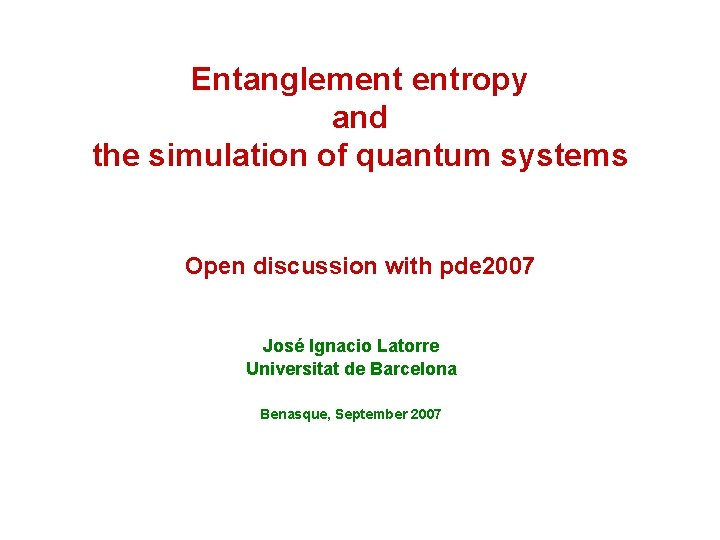Entanglement entropy and the simulation of quantum systems Open discussion with pde 2007 José Ignacio Latorre Universitat de Barcelona Benasque, September 2007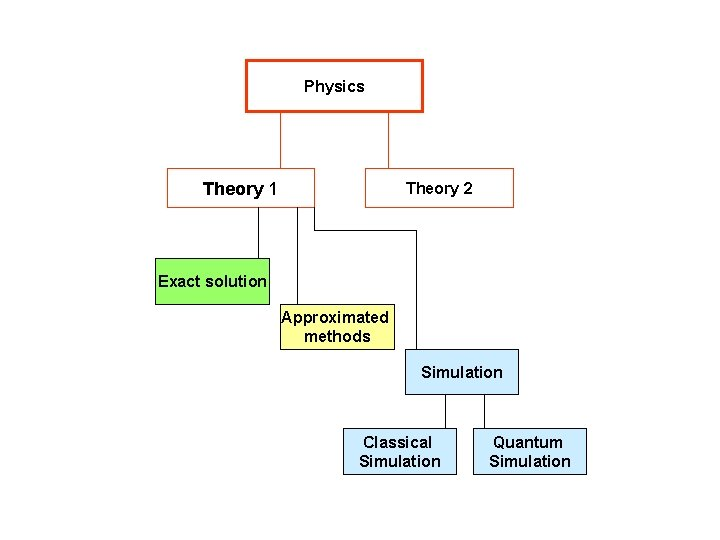Physics Theory 1 Theory 2 Exact solution Approximated methods Simulation Classical Simulation Quantum SimulationIntroduction Classical Theory • Classical simulation • Quantum simulation Classical computer ? Quantum Mechanics • Classical simulation • Quantum simulation Quantum computer Classical simulation of Quantum Mechanics is related to our ability to support large entanglement Classical simulation may be enough to handle e. g. ground states: MPS, PEPS, MERA Quantum simulation needed for time evolution of quantum systems and for non-local HamiltoniansIntroduction Is it possible to classically simulate faithfully a quantum system? Quantum Ising model represent evolve readIntroduction The lowest eigenvalue state carries a large superposition of product states Ex. n=3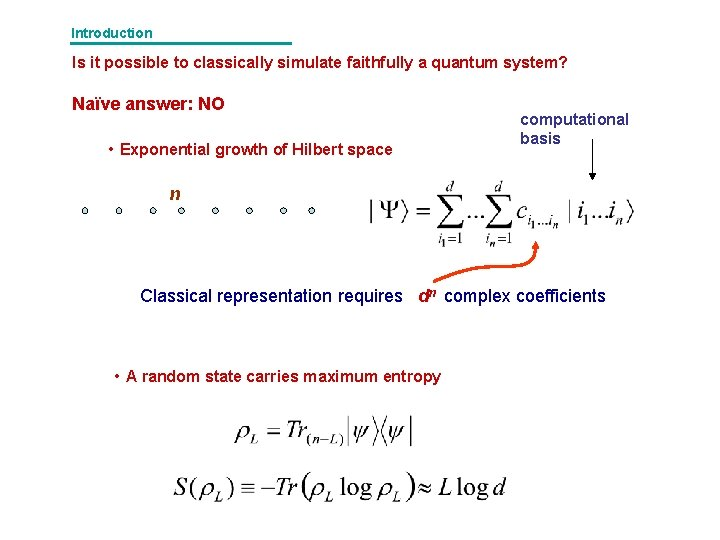Introduction Is it possible to classically simulate faithfully a quantum system? Naïve answer: NO • Exponential growth of Hilbert space computational basis n Classical representation requires dn complex coefficients • A random state carries maximum entropy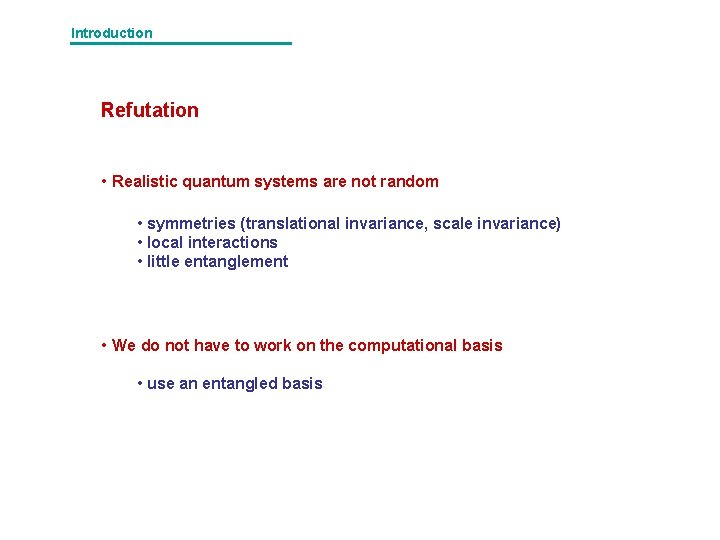Introduction Refutation • Realistic quantum systems are not random • symmetries (translational invariance, scale invariance) • local interactions • little entanglement • We do not have to work on the computational basis • use an entangled basis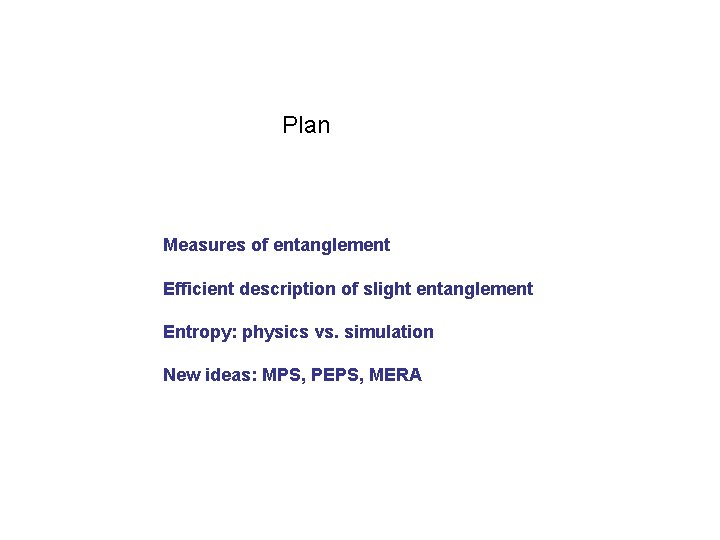Plan Measures of entanglement Efficient description of slight entanglement Entropy: physics vs. simulation New ideas: MPS, PEPS, MERA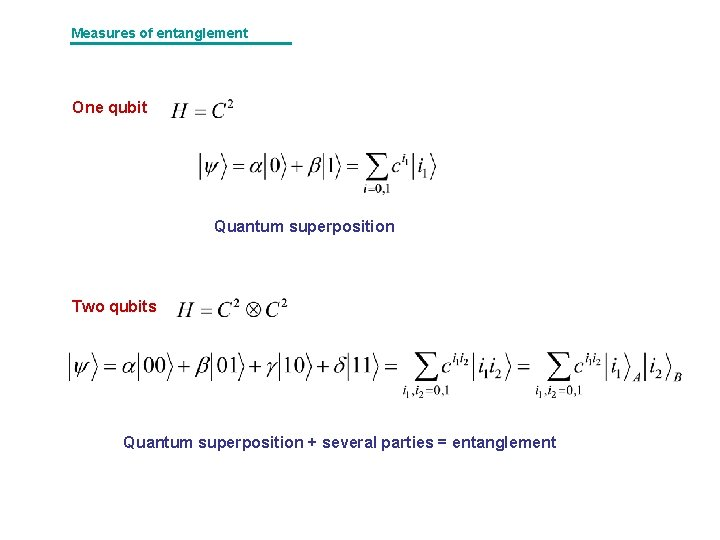Measures of entanglement One qubit Quantum superposition Two qubits Quantum superposition + several parties = entanglement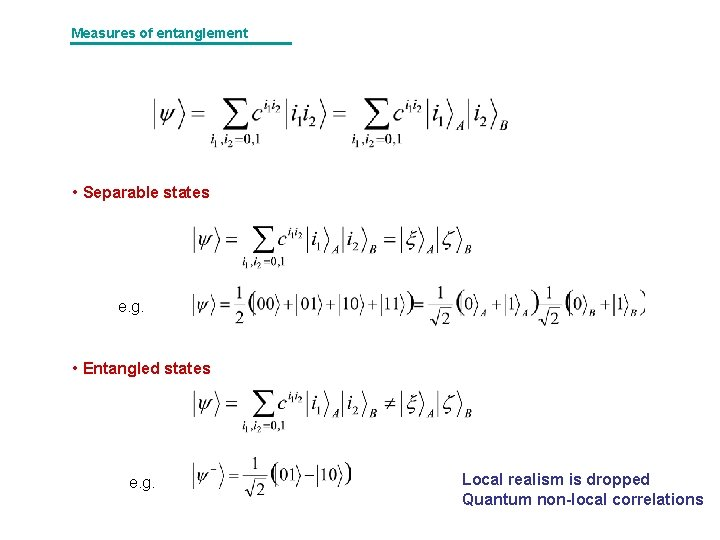Measures of entanglement • Separable states e. g. • Entangled states e. g. Local realism is dropped Quantum non-local correlations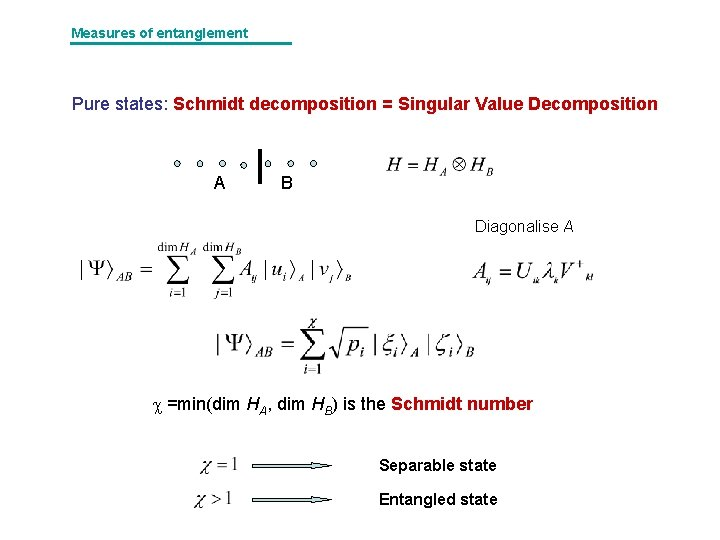Measures of entanglement Pure states: Schmidt decomposition = Singular Value Decomposition A B Diagonalise A =min(dim HA, dim HB) is the Schmidt number Separable state Entangled stateMeasures of entanglement Von Neumann entropy of the reduced density matrix Product state large e-bit large Very entangled state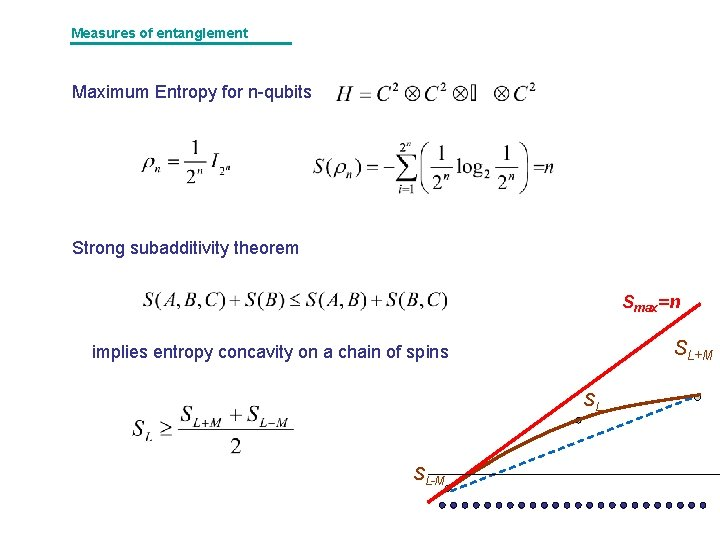Measures of entanglement Maximum Entropy for n-qubits Strong subadditivity theorem Smax=n SL+M implies entropy concavity on a chain of spins SL SL-M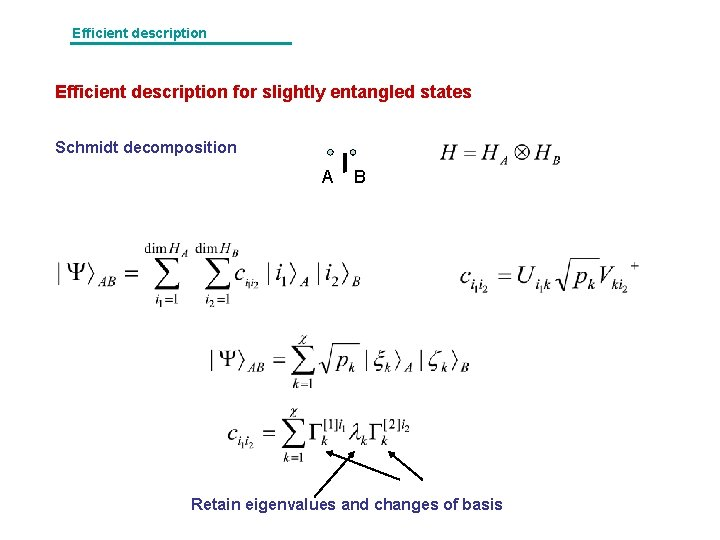Efficient description for slightly entangled states Schmidt decomposition A B Retain eigenvalues and changes of basis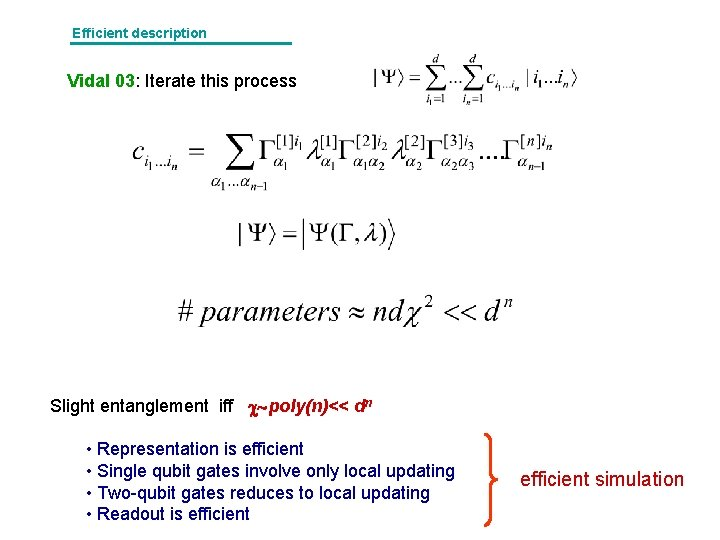Efficient description Vidal 03: Iterate this process Slight entanglement iff poly(n)<< dn • Representation is efficient • Single qubit gates involve only local updating • Two-qubit gates reduces to local updating • Readout is efficient simulationEfficient description Graphic representation of a MPS Efficient computation of scalar products operations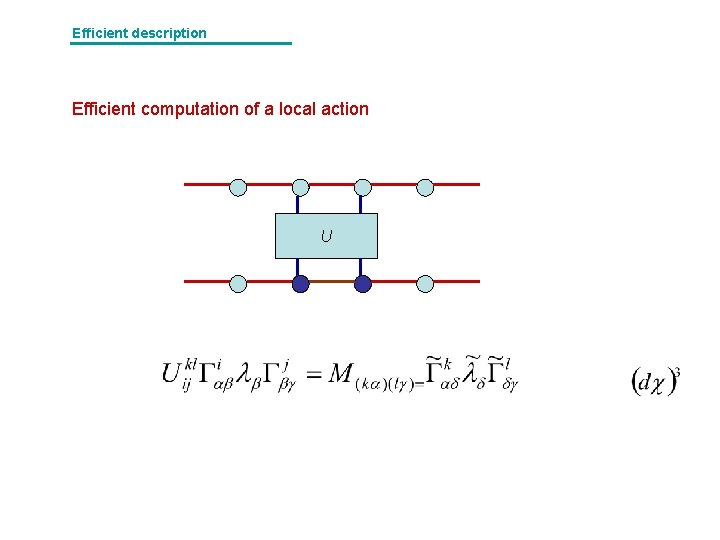Efficient description Efficient computation of a local action UEfficient description Matrix Product States i α canonical form PVWC 06 Approximate physical states with a finite MPS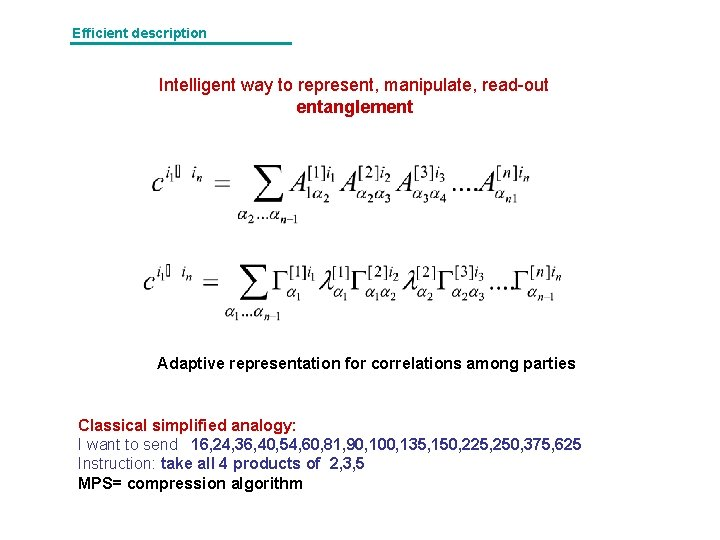Efficient description Intelligent way to represent, manipulate, read-out entanglement Adaptive representation for correlations among parties Classical simplified analogy: I want to send 16, 24, 36, 40, 54, 60, 81, 90, 100, 135, 150, 225, 250, 375, 625 Instruction: take all 4 products of 2, 3, 5 MPS= compression algorithmEfficient description Spin-off: Image compression | i|2 i 2, 1 i 11 105| i 2=1 i 2=2 i 1=1 i 2=3 i 2=4 i 1=3 i 1=2 RG addressing i 1=4 level of grey pixel addressEfficient description QPEG • Read image by blocks • Fourier transform • RG address and fill • Set compression level: • Find optimal • gzip (lossless, entropic compression) • (define discretize Γ’s to improve gzip) • diagonal organize the frequencies and use 1 d RG • work with diferences to a prefixed table =1 PSNR=17 =4 PSNR=25 Max = 81 =8 PSNR=31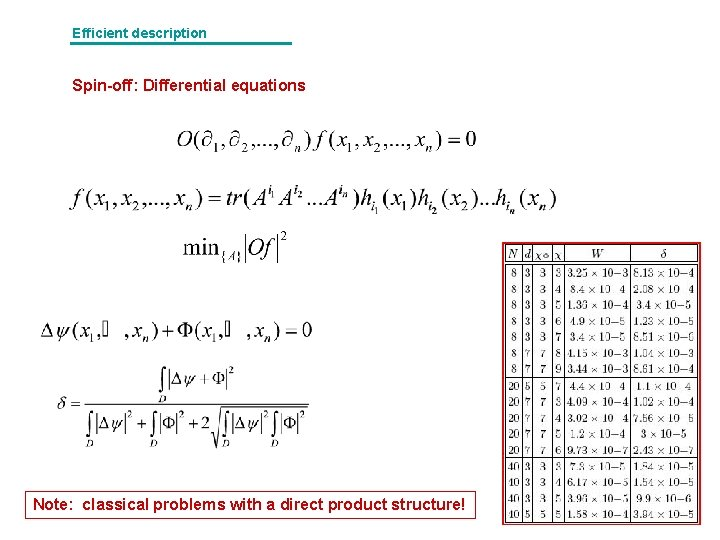Efficient description Spin-off: Differential equations Note: classical problems with a direct product structure!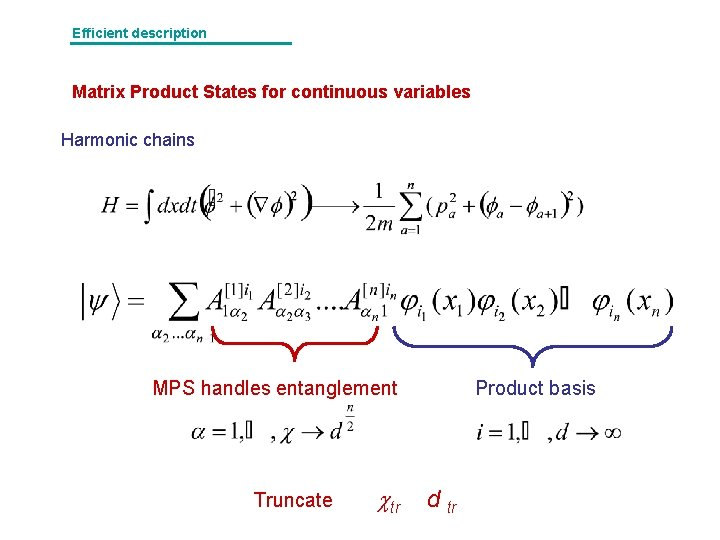Efficient description Matrix Product States for continuous variables Harmonic chains MPS handles entanglement Truncate tr Product basis d tr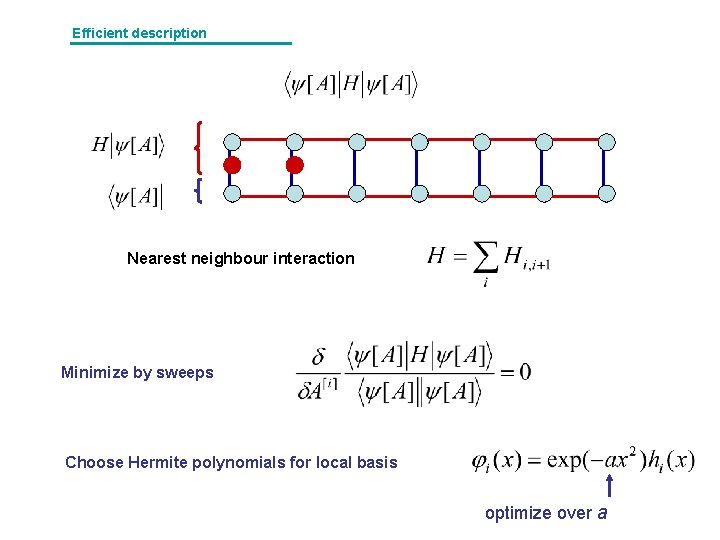Efficient description Nearest neighbour interaction Minimize by sweeps Choose Hermite polynomials for local basis optimize over a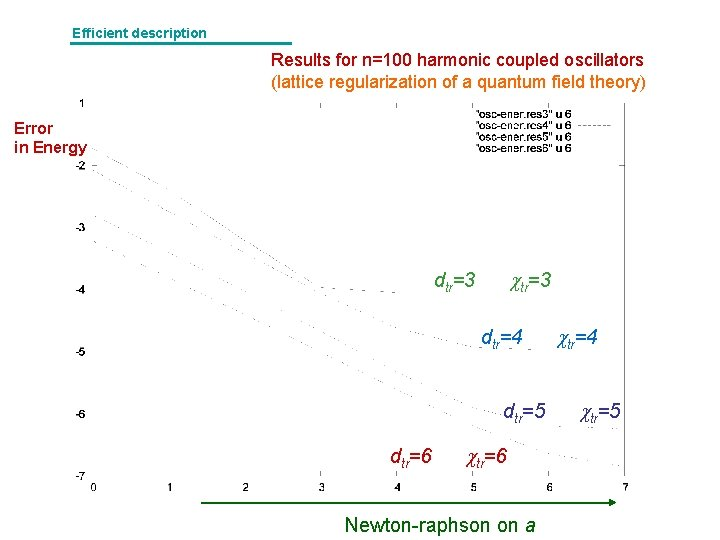Efficient description Results for n=100 harmonic coupled oscillators (lattice regularization of a quantum field theory) Error in Energy tr=3 dtr=4 dtr=5 dtr=6 Newton-raphson on a tr=4 tr=5Physics vs. simulation Back to the central idea: entanglement support Success of MPS will depend on how much entanglement is present in the physical state Physics If Simulation MPS is in very bad shape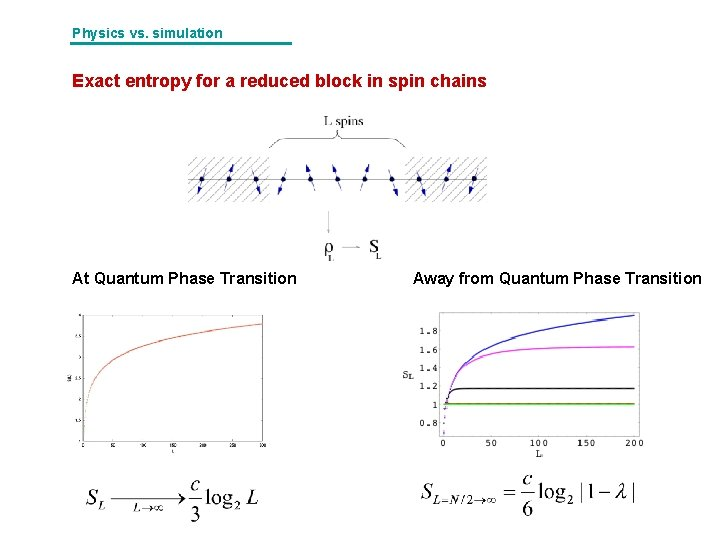Physics vs. simulation Exact entropy for a reduced block in spin chains At Quantum Phase Transition Away from Quantum Phase TransitionPhysics vs. simulation Maximum entropy support for MPS Maximum supported entanglementPhysics vs. simulation Faithfullness = Entanglement support MPS Spin chains Spin networks PEPS Area law Computations of entropies are no longer academic exercises but limits on simulations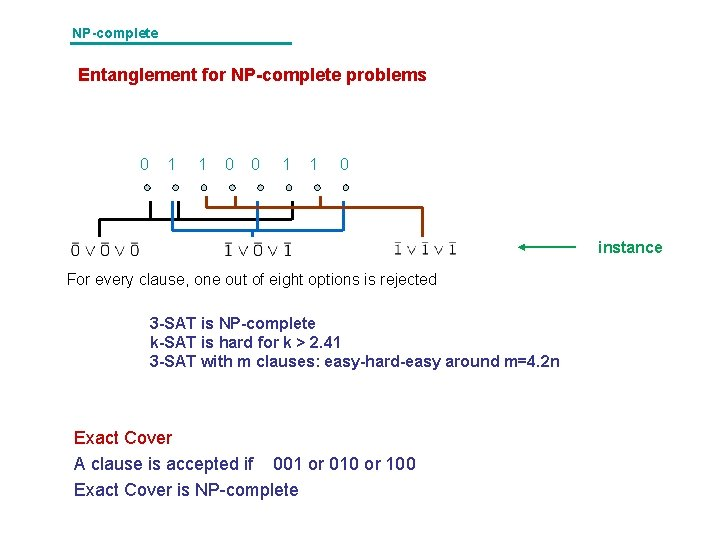NP-complete Entanglement for NP-complete problems 0 1 1 0 instance For every clause, one out of eight options is rejected 3 -SAT is NP-complete k-SAT is hard for k > 2. 41 3 -SAT with m clauses: easy-hard-easy around m=4. 2 n Exact Cover A clause is accepted if 001 or 010 or 100 Exact Cover is NP-complete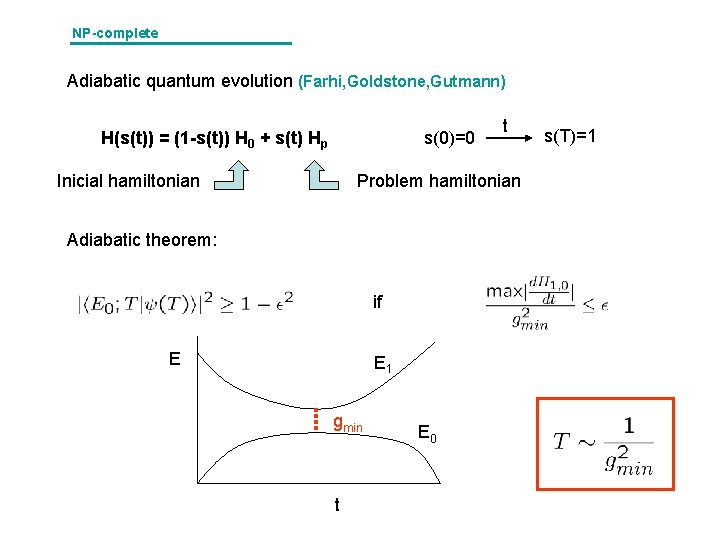NP-complete Adiabatic quantum evolution (Farhi, Goldstone, Gutmann) H(s(t)) = (1 -s(t)) H 0 + s(t) Hp s(0)=0 Inicial hamiltonian t Problem hamiltonian Adiabatic theorem: if E E 1 gmin t E 0 s(T)=1NP-complete Adiabatic quantum evolution for exact cover |0> |1> |1> |0> |1> (|0>+|1>)…. (|0>+|1>) NP problem as a non-local two-body hamiltonian!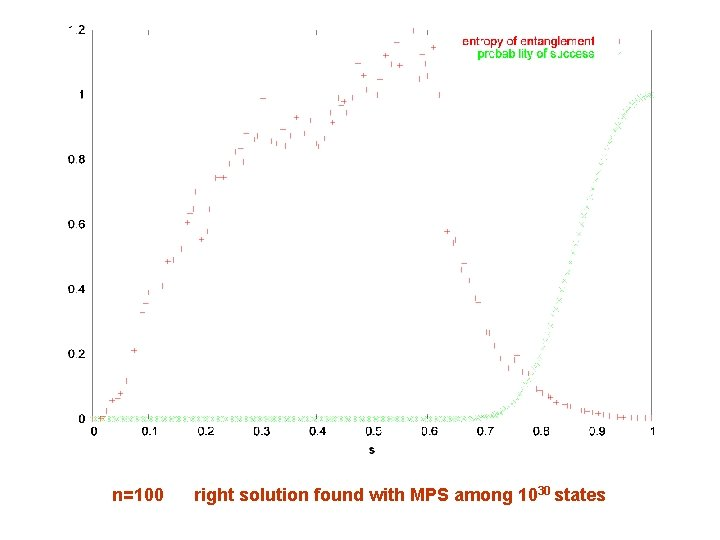n=100 right solution found with MPS among 1030 statesPhysics vs. simulation Non-critical spin chains S ~ ct Critical spin chains S ~ log 2 n Spin chains in d-dimensions S ~ nd-1/d Fermionic systems? S ~ n log 2 n NP-complete problems S ~. 1 n 3 -SAT Exact Cover Shor Factorization S~r~n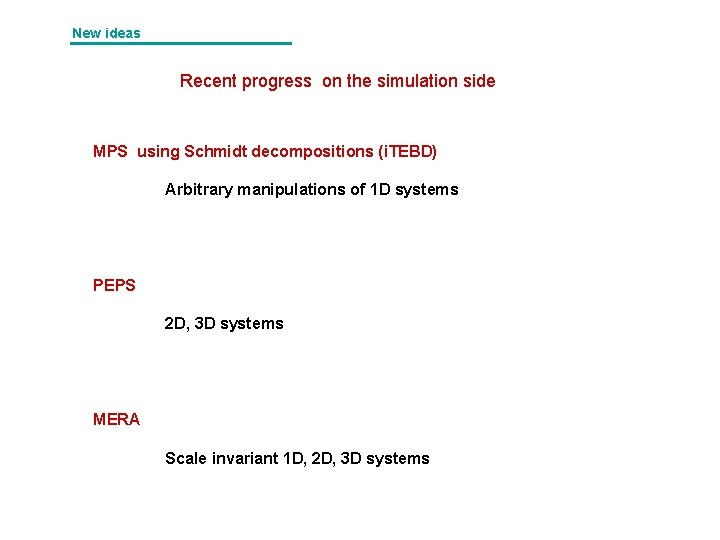New ideas Recent progress on the simulation side MPS using Schmidt decompositions (i. TEBD) Arbitrary manipulations of 1 D systems PEPS 2 D, 3 D systems MERA Scale invariant 1 D, 2 D, 3 D systemsMPS 2. Euclidean evolution Non-unitary evolution entails loss of norm are sums of commuting pieces Trotter expansionMPS Ex: i. TEBD (infinite time-evolving block decimation) A B A A B even B A A odd Translational invariance is momentarily broken A B BMPS i) iii) iv)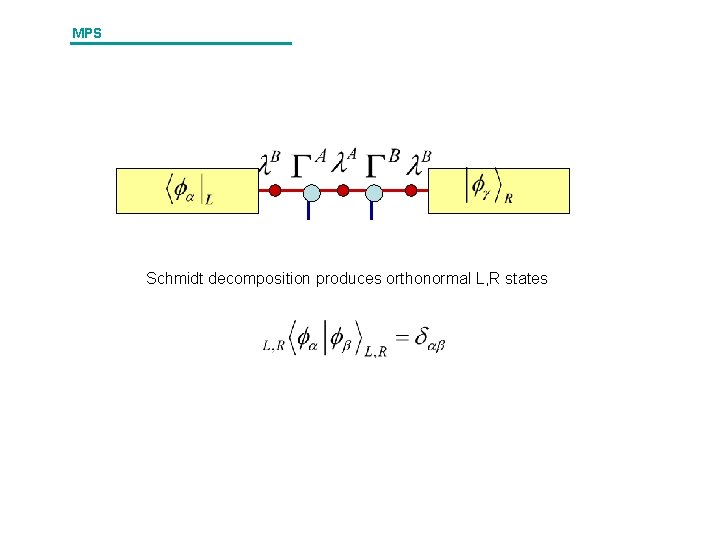MPS Schmidt decomposition produces orthonormal L, R states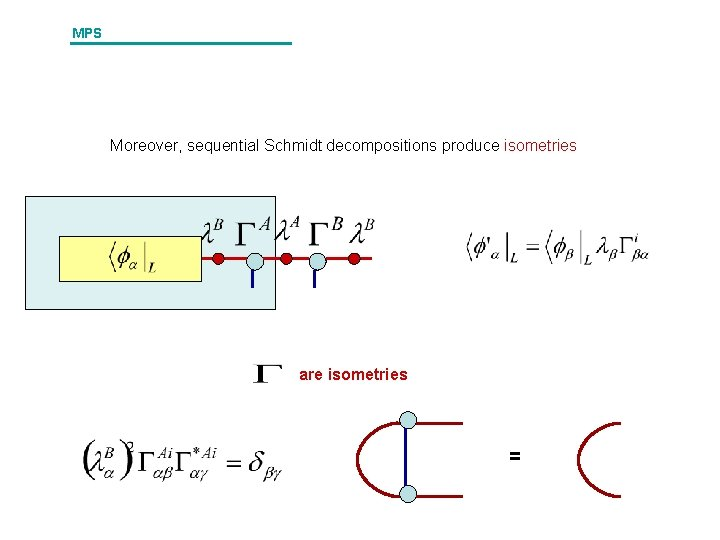MPS Moreover, sequential Schmidt decompositions produce isometries are isometries =MPS Read out Energy Entropy for half chain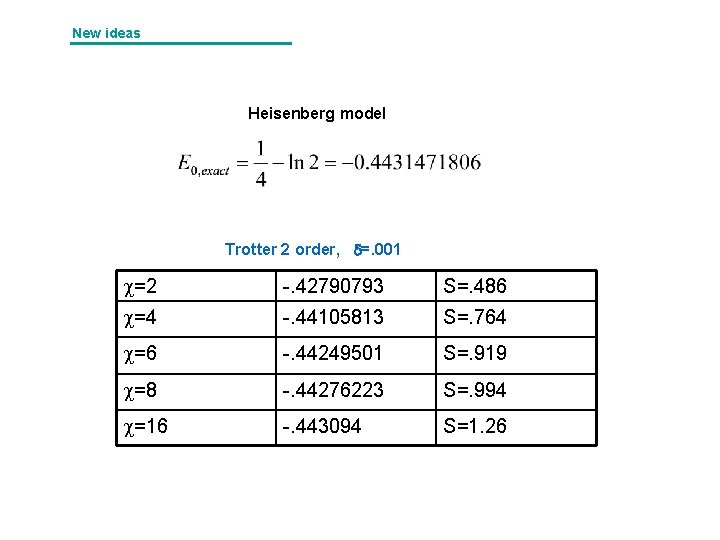New ideas Heisenberg model Trotter 2 order, =. 001 =2 -. 42790793 S=. 486 =4 -. 44105813 S=. 764 =6 -. 44249501 S=. 919 =8 -. 44276223 S=. 994 =16 -. 443094 S=1. 26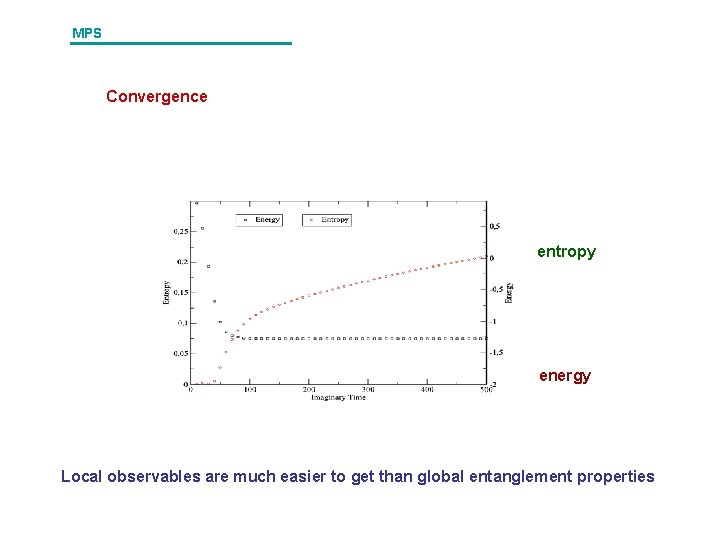MPS Convergence entropy energy Local observables are much easier to get than global entanglement properties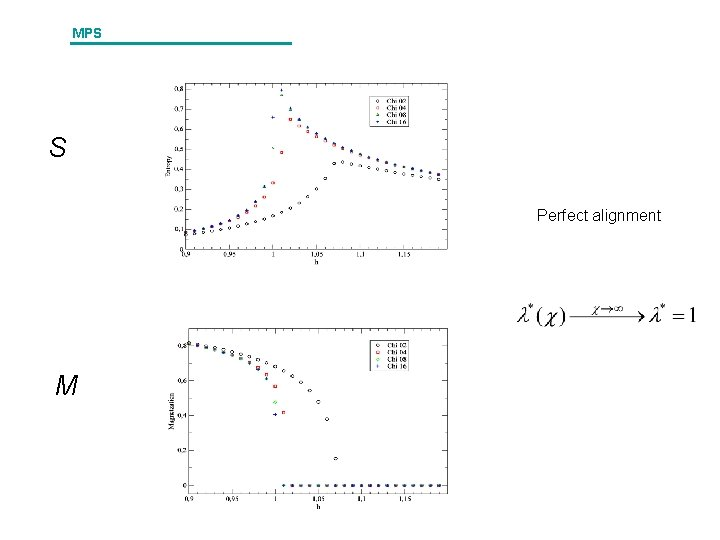MPS S Perfect alignment MNew ideas PEPS: Projected Entangled Pairs physical index ancillae Good: PEPS support an area law!! Bad: Contraction of PEPS is #P New results beat Monte Carlo simulationsPEPS Entropy is proportional to the boundary A B Contour A = L “Area law” Some violations of the area law have been identifiedPEPS Contraction of PEPS is #P Building physical PEPS would solve NP-complete problems As the contraction proceeds, the number of open indices grows as the area law 2 D seemed out of reach to any efficient representationPEPS E Yet, for translational invariant systems, it comes down to i. TEBD !! E becomes a non-unitary gate E E Comparable to quantum Monte Carlo?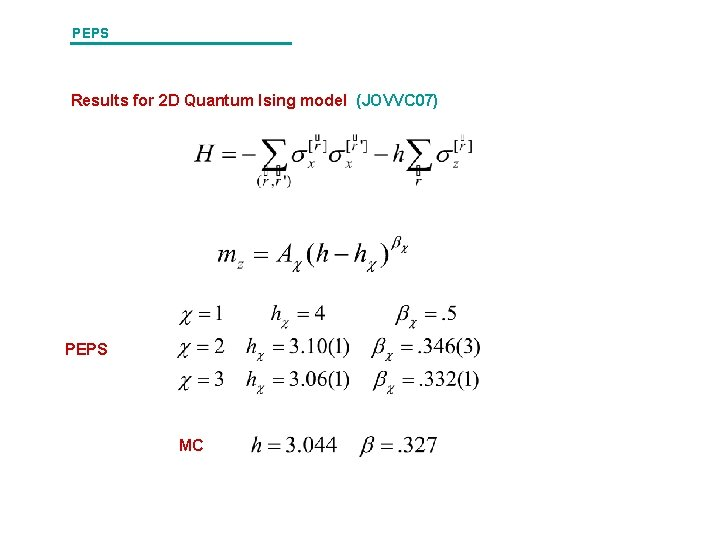PEPS Results for 2 D Quantum Ising model (JOVVC 07) PEPS MC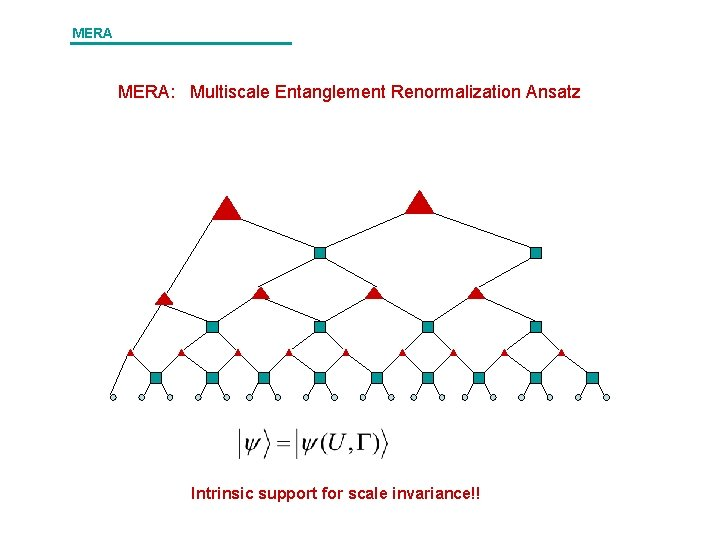MERA: Multiscale Entanglement Renormalization Ansatz Intrinsic support for scale invariance!!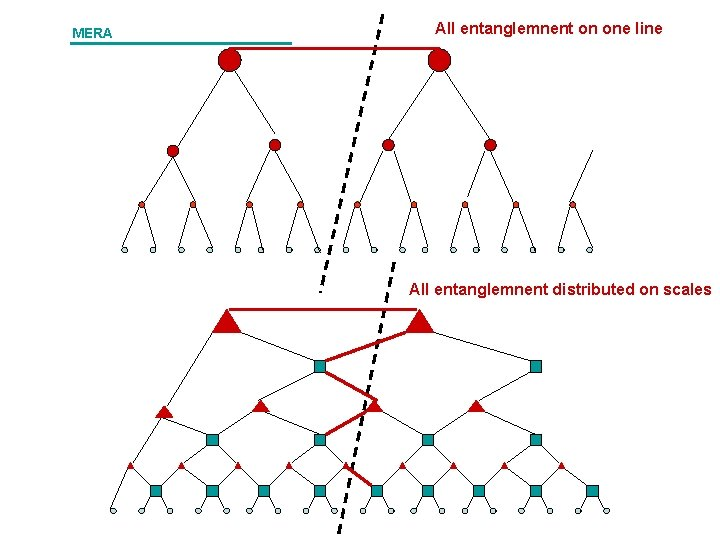MERA All entanglemnent on one line All entanglemnent distributed on scalesMERA Update U Contraction = Identity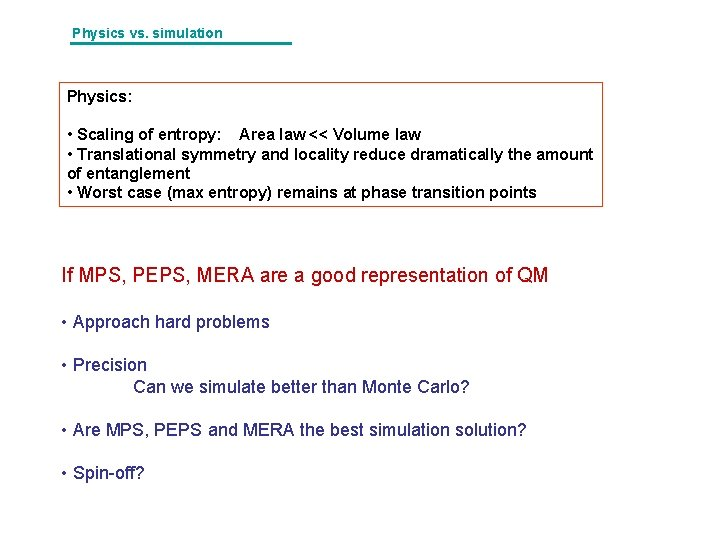Physics vs. simulation Physics: • Scaling of entropy: Area law << Volume law • Translational symmetry and locality reduce dramatically the amount of entanglement • Worst case (max entropy) remains at phase transition points If MPS, PEPS, MERA are a good representation of QM • Approach hard problems • Precision Can we simulate better than Monte Carlo? • Are MPS, PEPS and MERA the best simulation solution? • Spin-off?QMA Quantum Complexity Classes L is in QMA if there exists a fixed and a polynomial time verifier (V) such that What is the QMA-complete problem? Feynman idea (shaped by Kitaev)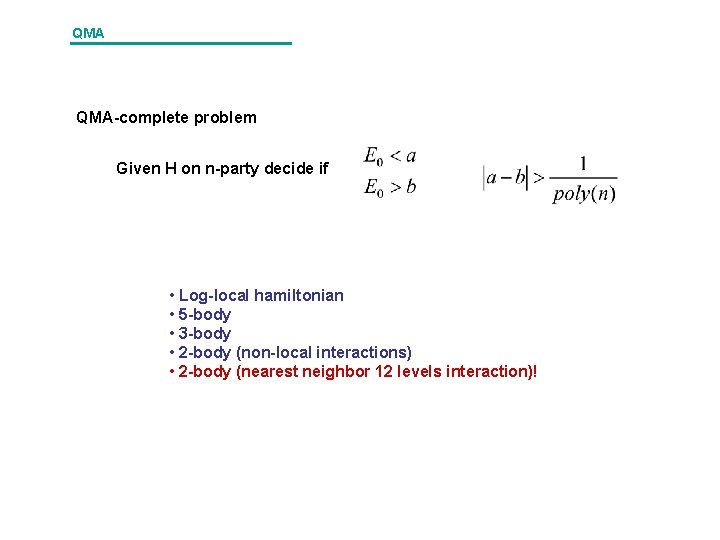QMA QMA-complete problem Given H on n-party decide if • Log-local hamiltonian • 5 -body • 3 -body • 2 -body (non-local interactions) • 2 -body (nearest neighbor 12 levels interaction)!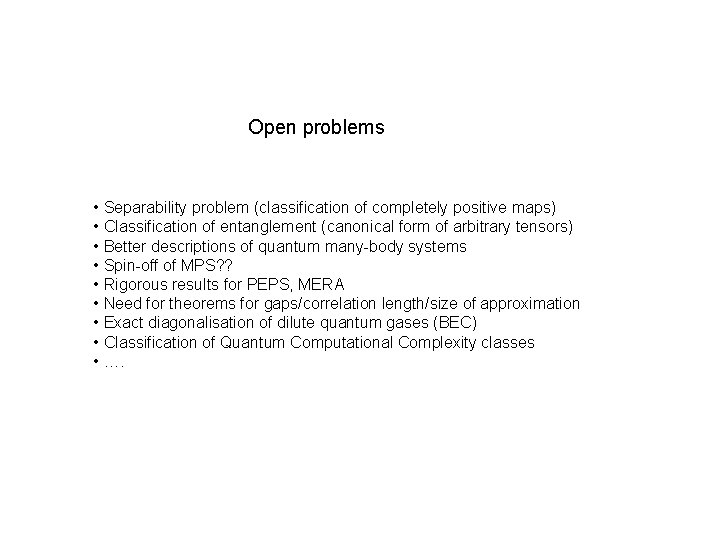Open problems • Separability problem (classification of completely positive maps) • Classification of entanglement (canonical form of arbitrary tensors) • Better descriptions of quantum many-body systems • Spin-off of MPS? ? • Rigorous results for PEPS, MERA • Need for theorems for gaps/correlation length/size of approximation • Exact diagonalisation of dilute quantum gases (BEC) • Classification of Quantum Computational Complexity classes • ….